## Generalization

The lexicographic construction may be extended to produce codes with desired characteristics using the generalized lexicographic construction, which we abbreviate as the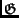-construction. The-construction replaces the lexicographically earliest'' heuristic used in building lexicodes with an arbitrary function. This allows us to generate arbitrary greedy codes in which various properties are grafted upon the good code parameters of the lexicodes.

Definition 1   The generalized lexicographic construction is initialized with a linear (n, k, d ) seed code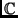and iteratively constructs the family of codes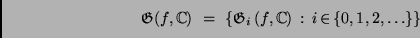using a mapping from codes to vectors:

 f ( . ) :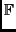q*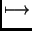v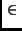q*. (1)

The construction follows the scheme:

•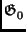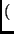f,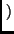is trivially the code;
•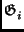f,is computed by adding to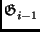f,the generator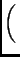1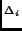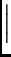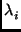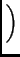(2)

where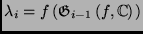and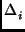is defined to be d minus the Hamming distance fromto the codef,.

We will call f ( . ) the generating mapping of the construction. The familiar lexicode family of minimum distance d is thus the simple special case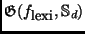, where we use the trivial seed coded{0d, 1d}. The code in Table 1 is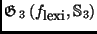, as can be verified by hand.

Despite its generality, there are many linear codes that cannot be constructed using a non-trivial application of the-construction. The code given by the following basis vectors is one such example:

1110000
0001111

For sake of simplicity we shall concern ourselves only with binary codes in the remainder of this paper, though the extension to q-ary codes is fairly straightforward.